Chapter 4 Class 11 Mathematical Induction
Concept wise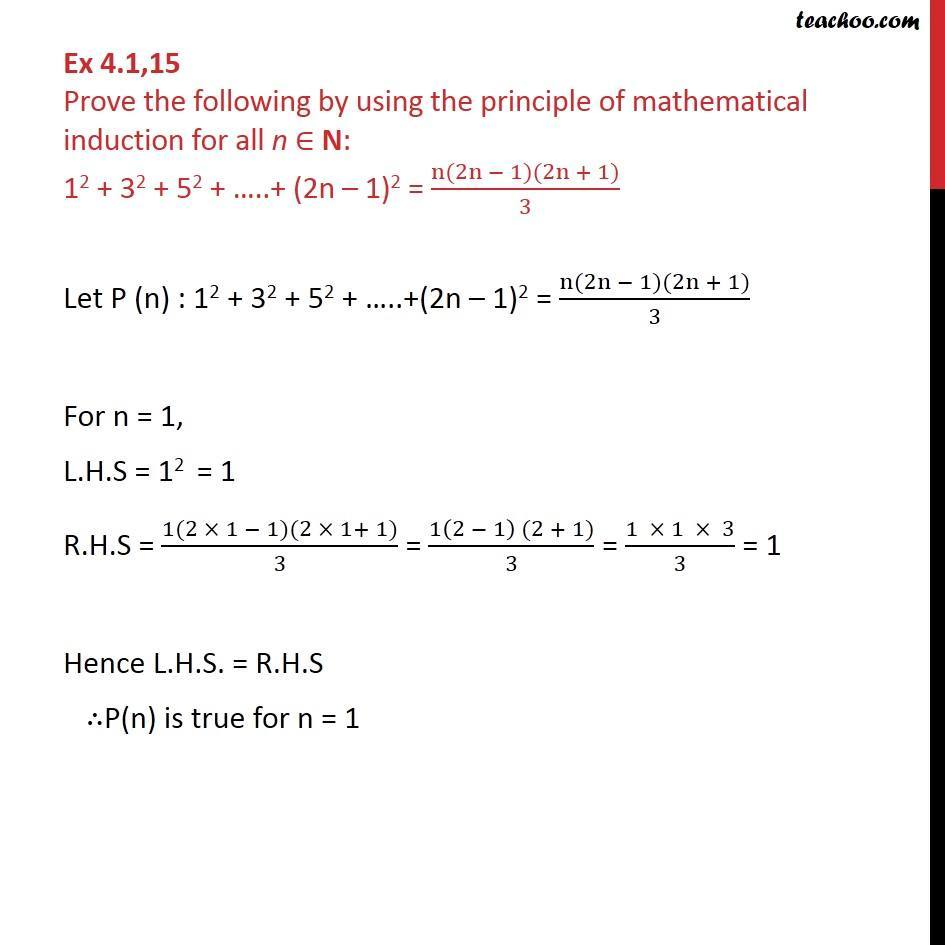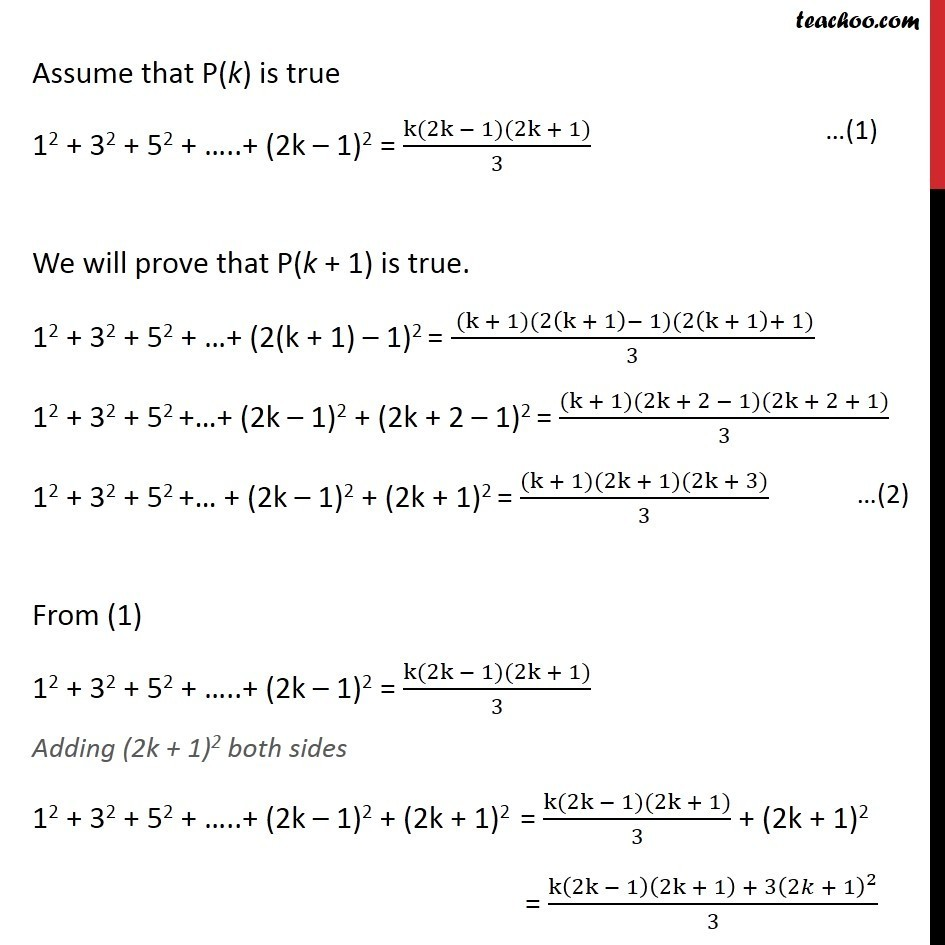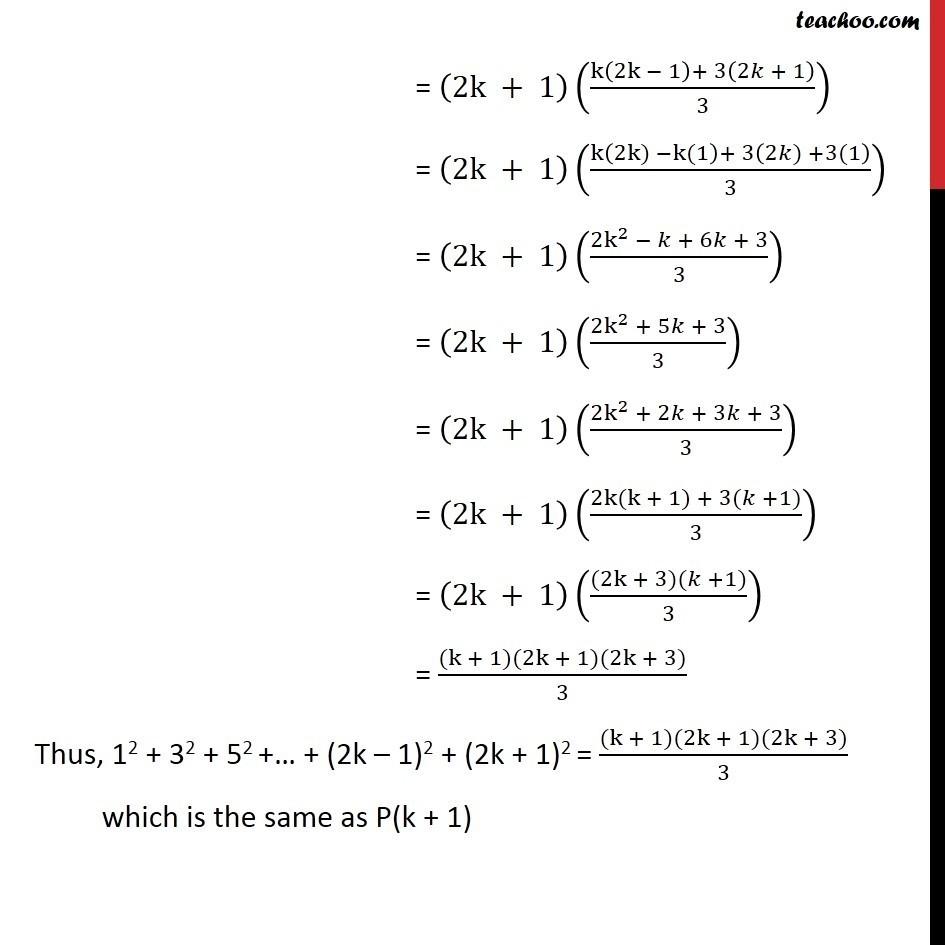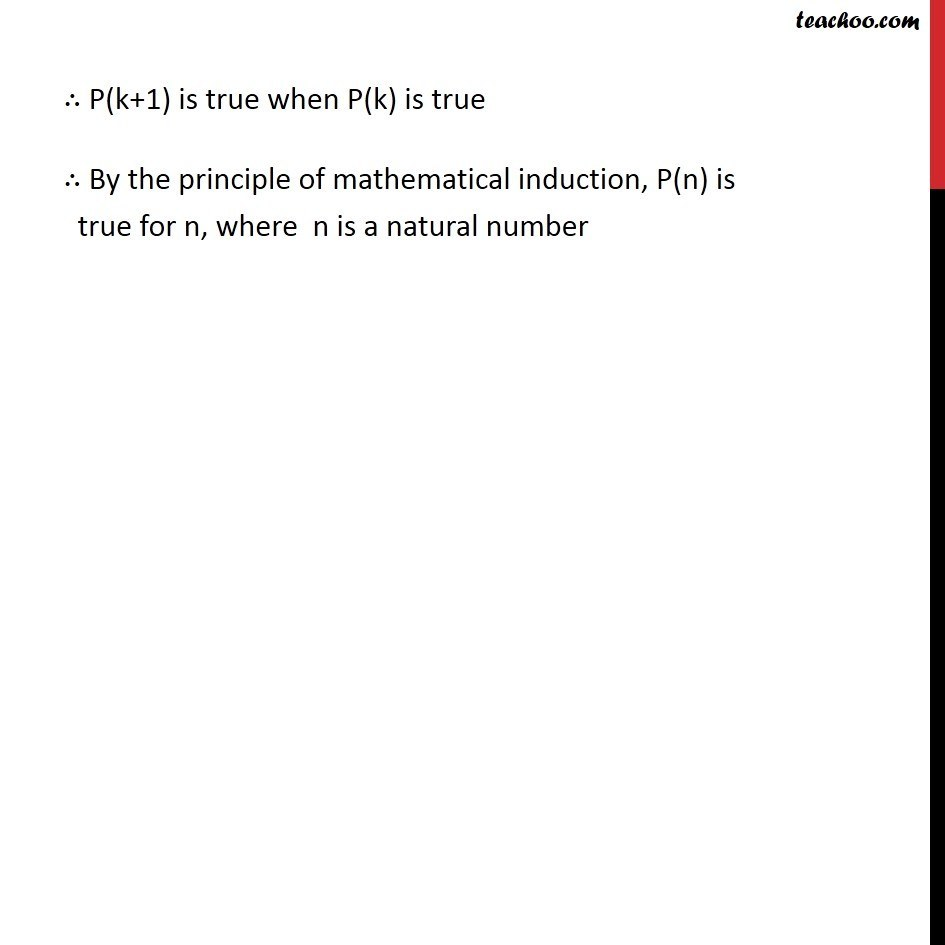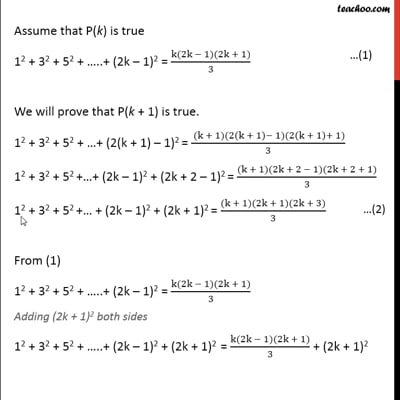This video is only available for Teachoo black users

Introducing your new favourite teacher - Teachoo Black, at only ₹83 per month

### Transcript

Ex 4.1,15 Prove the following by using the principle of mathematical induction for all n N: 12 + 32 + 52 + ..+ (2n 1)2 = (n(2n 1)(2n + 1))/3 Let P (n) : 12 + 32 + 52 + ..+(2n 1)2 = (n(2n 1)(2n + 1))/3 For n = 1, L.H.S = 12 = 1 R.H.S = (1(2 1 1)(2 1+ 1))/3 = (1(2 1) (2 + 1))/3 = (1 1 3)/3 = 1 Hence L.H.S. = R.H.S P(n) is true for n = 1 Assume that P(k) is true 12 + 32 + 52 + ..+ (2k 1)2 = (k(2k 1)(2k + 1))/3 We will prove that P(k + 1) is true. 12 + 32 + 52 + + (2(k + 1) 1)2 = ( (k + 1)(2(k + 1) 1)(2(k + 1)+ 1))/3 12 + 32 + 52 + + (2k 1)2 + (2k + 2 1)2 = ((k + 1)(2k + 2 1)(2k + 2 + 1))/3 12 + 32 + 52 + + (2k 1)2 + (2k + 1)2 = ((k + 1)(2k + 1)(2k + 3))/3 From (1) 12 + 32 + 52 + ..+ (2k 1)2 = (k(2k 1)(2k + 1))/3 Adding (2k + 1)2 both sides 12 + 32 + 52 + ..+ (2k 1)2 + (2k + 1)2 = (k(2k 1)(2k + 1))/3 + (2k + 1)2 = (k(2k 1)(2k + 1) + 3(2 + 1)^2)/3 = (2k + 1)((k(2k 1)+ 3(2 + 1))/3) = (2k + 1)((k(2k) k(1)+ 3(2 ) +3(1))/3) = (2k + 1)((2k^2 + 6 + 3)/3) = (2k + 1)((2k^2 + 5 + 3)/3) = (2k + 1)((2k^2 + 2 + 3 + 3)/3) = (2k + 1)((2k(k + 1) + 3( +1))/3) = (2k + 1)(((2k + 3)( +1))/3) = ((k + 1)(2k + 1)(2k + 3))/3 Thus, 12 + 32 + 52 + + (2k 1)2 + (2k + 1)2 = ((k + 1)(2k + 1)(2k + 3))/3 which is the same as P(k + 1) P(k+1) is true when P(k) is true By the principle of mathematical induction, P(n) is true for n, where n is a natural number